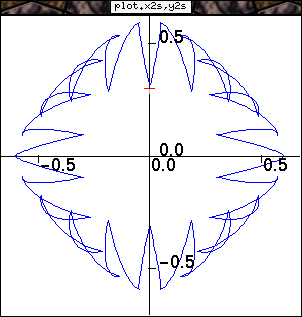# The plotline operator

Agat can be used like a plotter of functions (like gnuplot, but agat plots as soon as possible and thus enable an earlier display)

Here is an example of a function representation. This C code is of very low interest. It only sends some integers (1,2,3,...) to agat. Then agat computes values to plot. I's a bit artificial, in a more practical case the algorithm should send values to plot directly to the grapical operators of agat. So this code is here to show that the agat embeded langage allows some complex constructs.

## The C code

```#include <stdio.h>
#include <math.h>
#include "libAGAT.h"

int main(argc, argv)
int             argc;
char          **argv;
{
int             i,j;
int             nbn;

if (argc<1)
nbn=1000;
else
nbn=atoi(argv);
agatSendInt("nb",nbn);
for (i = 1; i <= nbn; i++) {
if (i%100==0)
agatSendInt("reinit",1);
else
agatSendInt("reinit",0);
agatSendInt("i",i);
}
}
```

## The Agat code

```let PI= atan(1)*4;

let t2d= map{step}(i,+-> step*i
|*,nb^-> step := 2 * PI / nb,
null
) i,nb;

let x2s= map(t-> sin(t)*(0.3+abs(0.3*cos(10*t))))t2d;
let y2s= map(t-> cos(t)*(0.3+abs(0.3*sin(10*t))))t2d;

plotaxeline(x2s,y2s);
```

## The result of the execution